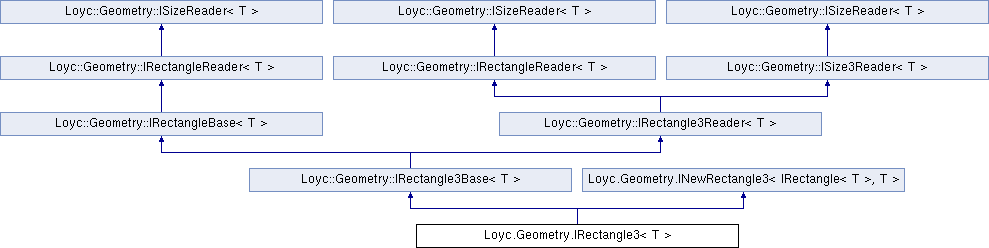Enhanced C# Language of your choice: library documentation
Loyc.Geometry.IRectangle3< T > Interface Template Reference

Represents a mutable 3D rectangle. More...

Source file:
Inheritance diagram for Loyc.Geometry.IRectangle3< T >:Remarks

Represents a mutable 3D rectangle.

This interface is separated into two bases, IRectangle3Base<T> and INewRectangle3<R,T>, for the same reason that IPoint<T>'s coordinates are divided into IPointBase<T> and INewPoint<P,T>, as explained in the documentation of IPoint<T>.Properties inherited from Loyc.Geometry.IRectangleReader< T >
X1 [get]

Y1 [get]

X2 [get]

Y2 [get]Properties inherited from Loyc.Geometry.ISizeReader< T >
Width [get]
Gets the width of a rectangle (the difference between X coordinates). More...

Height [get]
Gets the height of a rectangle (the difference between Y coordinates). More...Properties inherited from Loyc.Geometry.IRectangle3Reader< T >
Z1 [get]

Z2 [get]Properties inherited from Loyc.Geometry.ISize3Reader< T >
Depth [get]
Gets the depth of a rectangle (the difference between Z coordinates). More...Public Member Functions inherited from Loyc.Geometry.IRectangle3Base< T >
void SetZAndDepth (T z, T depth)

void SetZRange (T z1, T z2)Public Member Functions inherited from Loyc.Geometry.IRectangleBase< T >
void SetXAndWidth (T x, T width)

void SetYAndHeight (T y, T height)

void SetXRange (T x1, T x2)

void SetYRange (T x1, T x2)Public Member Functions inherited from Loyc.Geometry.INewRectangle3< IRectangle< T >, T >
Rect NewRect (T x, T y, T z, T width, T height, T depth)

Rect NewRange (T x1, T y1, T z1, T x2, T y2, T z2)